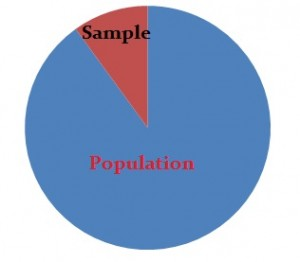Share on

## What is Probability Sampling?Sampling takes on two forms in statistics: probability sampling and non-probability sampling:

• Probability sampling uses random sampling techniques to create a sample. For each element in the sample, the probability is known and non-zero. In principal, every element of the population has the same chance at being included in the sample. This is a achieved with a sampling frame.
• Non-probability sampling techniques use non-random processes like researcher judgment or convenience sampling. The probability of being selected for the sample is unknown.

Probability sampling is based on the fact that every member of a population has a known and equal chance of being selected. For example, if you had a population of 100 people, each person would have odds of 1 out of 100 of being chosen. With non-probability sampling, those odds are not equal. For example, a person might have a better chance of being chosen if they live close to the researcher or have access to a computer. Probability sampling gives you the best chance to create a sample that is truly representative of the population.

As a rule of thumb, your sample size should be over about 30. If you have a small sample, you may need to try one of the non-probability sampling techniques instead.

Watch the video for an overview of probability sampling:

## Types of Probability Sampling

• Simple random sampling is a completely random method of selecting subjects. These can include assigning numbers to all subjects and then using a random number generator to choose random numbers. Classic ball and urn experiments are another example of this process (assuming the balls are sufficiently mixed). The members whose numbers are chosen are included in the sample.
• Stratified Random Sampling involves splitting subjects into mutually exclusive groups and then using simple random sampling to choose members from groups.
• Systematic Sampling means that you choose every “nth” participant from a complete list. For example, you could choose every 10th person listed.
• Cluster Random Sampling is a way to randomly select participants from a list that is too large for simple random sampling. For example, if you wanted to choose 1000 participants from the entire population of the U.S., it is likely impossible to get a complete list of everyone. Instead, the researcher randomly selects areas (i.e. cities or counties) and randomly selects from within those boundaries.
• Multi-Stage Random sampling uses a combination of techniques.

Each probability sampling method has its own unique advantages and disadvantages. In general, probability sampling minimized the risk of systematic bias. This means that you are reducing the risk of over- or under-representation--ensuring your results are representative of the population.

Using probability sampling also means that you can use statistical techniques like confidence intervals and margins of error to validate your results.

• Cluster sampling: convenience and ease of use.
• Simple random sampling: creates samples that are highly representative of the population.
• Stratified random sampling: creates strata or layers that are highly representative of strata or layers in the population.
• Systematic sampling: creates samples that are highly representative of the population, without the need for a random number generator.

• Cluster sampling: might not work well if unit members are not homogeneous (i.e. if they are different from each other).
• Simple random sampling: tedious and time consuming, especially when creating larger samples.
• Stratified random sampling: tedious and time consuming, especially when creating larger samples.
• Systematic sampling: not as random as simple random sampling,

## References

Cook, T. (2005). Introduction to Statistical Methods for Clinical Trials (Chapman & Hall/CRC Texts in Statistical Science) 1st Edition. Chapman and Hall/CRC
Everitt, B. S.; Skrondal, A. (2010), The Cambridge Dictionary of Statistics, Cambridge University Press.
Levine, D. (2014). Even You Can Learn Statistics and Analytics: An Easy to Understand Guide to Statistics and Analytics 3rd Edition. Pearson FT Press

CITE THIS AS:
Stephanie Glen. "Probability Sampling: Definition,Types, Advantages and Disadvantages" From StatisticsHowTo.com: Elementary Statistics for the rest of us! https://www.statisticshowto.com/probability-and-statistics/sampling-in-statistics/probability-sampling/
---------------------------------------------------------------------------Need help with a homework or test question? With Chegg Study, you can get step-by-step solutions to your questions from an expert in the field. Your first 30 minutes with a Chegg tutor is free!